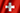# Clima Blatten (Lötschen)

## Temperatura, Precipitación

precipitación Durchschnittliche Maximaltemperatur Durchschnittliche Minimaltemperatur
mm °C °C
dic.hidden diciembre 120 0 -7
ene. enero 95 0 -9
feb. febrero 80 2 -9
mar. marzo 75 5 -5
abr. abril 60 9 -1
may. mayo 95 14 3
jun. junio 90 18 5
jul. julio 95 20 7
ago. agosto 100 20 7
sep. septiembre 70 16 4
oct. octubre 90 12 1
nov. noviembre 95 5 -4
dic. diciembre 120 0 -7
ene.hidden enero 95 0 -9

## enero

fuente de datos: MeteoSchweiz

For the month of enero, average values for station „Blatten (Lötschen)“ were calculated from climate data measured between the years 1991 and 2020.

## febrero

fuente de datos: MeteoSchweiz

For the month of febrero, average values for station „Blatten (Lötschen)“ were calculated from climate data measured between the years 1991 and 2020.

## marzo

fuente de datos: MeteoSchweiz

For the month of marzo, average values for station „Blatten (Lötschen)“ were calculated from climate data measured between the years 1991 and 2020.

## abril

fuente de datos: MeteoSchweiz

For the month of abril, average values for station „Blatten (Lötschen)“ were calculated from climate data measured between the years 1991 and 2020.

## mayo

fuente de datos: MeteoSchweiz

For the month of mayo, average values for station „Blatten (Lötschen)“ were calculated from climate data measured between the years 1991 and 2020.

## junio

fuente de datos: MeteoSchweiz

For the month of junio, average values for station „Blatten (Lötschen)“ were calculated from climate data measured between the years 1991 and 2020.

## julio

fuente de datos: MeteoSchweiz

For the month of julio, average values for station „Blatten (Lötschen)“ were calculated from climate data measured between the years 1991 and 2020.

## agosto

fuente de datos: MeteoSchweiz

For the month of agosto, average values for station „Blatten (Lötschen)“ were calculated from climate data measured between the years 1991 and 2020.

## septiembre

fuente de datos: MeteoSchweiz

For the month of septiembre, average values for station „Blatten (Lötschen)“ were calculated from climate data measured between the years 1991 and 2020.

## octubre

fuente de datos: MeteoSchweiz

For the month of octubre, average values for station „Blatten (Lötschen)“ were calculated from climate data measured between the years 1991 and 2020.

## noviembre

fuente de datos: MeteoSchweiz

For the month of noviembre, average values for station „Blatten (Lötschen)“ were calculated from climate data measured between the years 1991 and 2020.

## diciembre

fuente de datos: MeteoSchweiz

For the month of diciembre, average values for station „Blatten (Lötschen)“ were calculated from climate data measured between the years 1991 and 2020.

## Clima

What is the hottest day of the year? Best chance of the least amount of rain? Find answers in our climate data.

## Blatten (Lötschen)

 paísSuiza canton Kanton Wallis Altura 1538 m.s.n.m. coordenadas 46° 25.2' N 7° 49.2' E